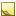﻿ Convolution.Divisor PropertyDivision factor.

Namespace:  Accord.Imaging.Filters
Assembly:  Accord.Imaging (in Accord.Imaging.dll) Version: 3.8.0Syntax
`public int Divisor { get; set; }`

#### Property Value

Type: Int32Exceptions
ExceptionCondition
ArgumentExceptionDivisor can not be equal to zero.Remarks

The value is used to divide convolution - weighted sum of pixels is divided by this value.Note
The value may be calculated automatically in the case if constructor with one parameter is used (Convolution(Int32)).See Also